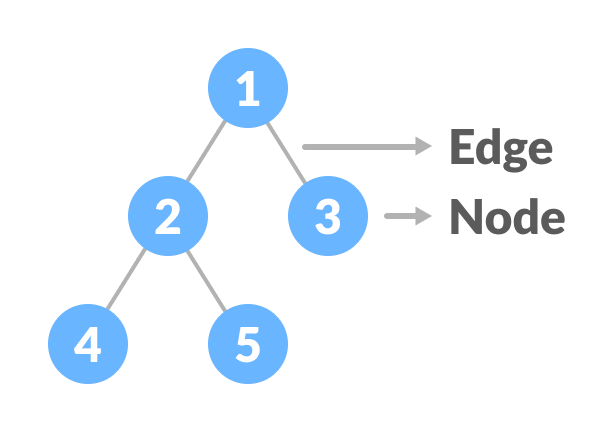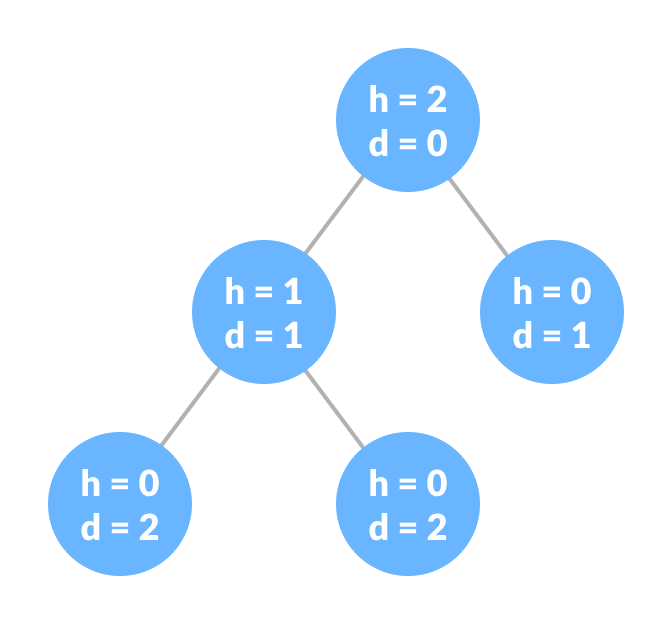# Tree Data Structure

In this tutorial, you will learn about tree data structure. Also, you will learn about different types of trees and the terminologies used in tree.

A tree is a nonlinear hierarchical data structure that consists of nodes connected by edges.

## Why Tree Data Structure?

Other data structures such as arrays, linked list, stack, and queue are linear data structures that store data sequentially. In order to perform any operation in a linear data structure, the time complexity increases with the increase in the data size. But, it is not acceptable in today's computational world.

Different tree data structures allow quicker and easier access to the data as it is a non-linear data structure.

## Tree Terminologies

### Node

A node is an entity that contains a key or value and pointers to its child nodes.

The last nodes of each path are called leaf nodes or external nodes that do not contain a link/pointer to child nodes.

The node having at least a child node is called an internal node.

### Edge

It is the link between any two nodes.Nodes and edges of a tree

### Root

It is the topmost node of a tree.

### Height of a Node

The height of a node is the number of edges from the node to the deepest leaf (ie. the longest path from the node to a leaf node).

### Depth of a Node

The depth of a node is the number of edges from the root to the node.

### Height of a Tree

The height of a Tree is the height of the root node or the depth of the deepest node.Height and depth of each node in a tree

### Degree of a Node

The degree of a node is the total number of branches of that node.

### Forest

A collection of disjoint trees is called a forest.

You can create a forest by cutting the root of a tree.

## Tree Traversal

In order to perform any operation on a tree, you need to reach to the specific node. The tree traversal algorithm helps in visiting a required node in the tree.